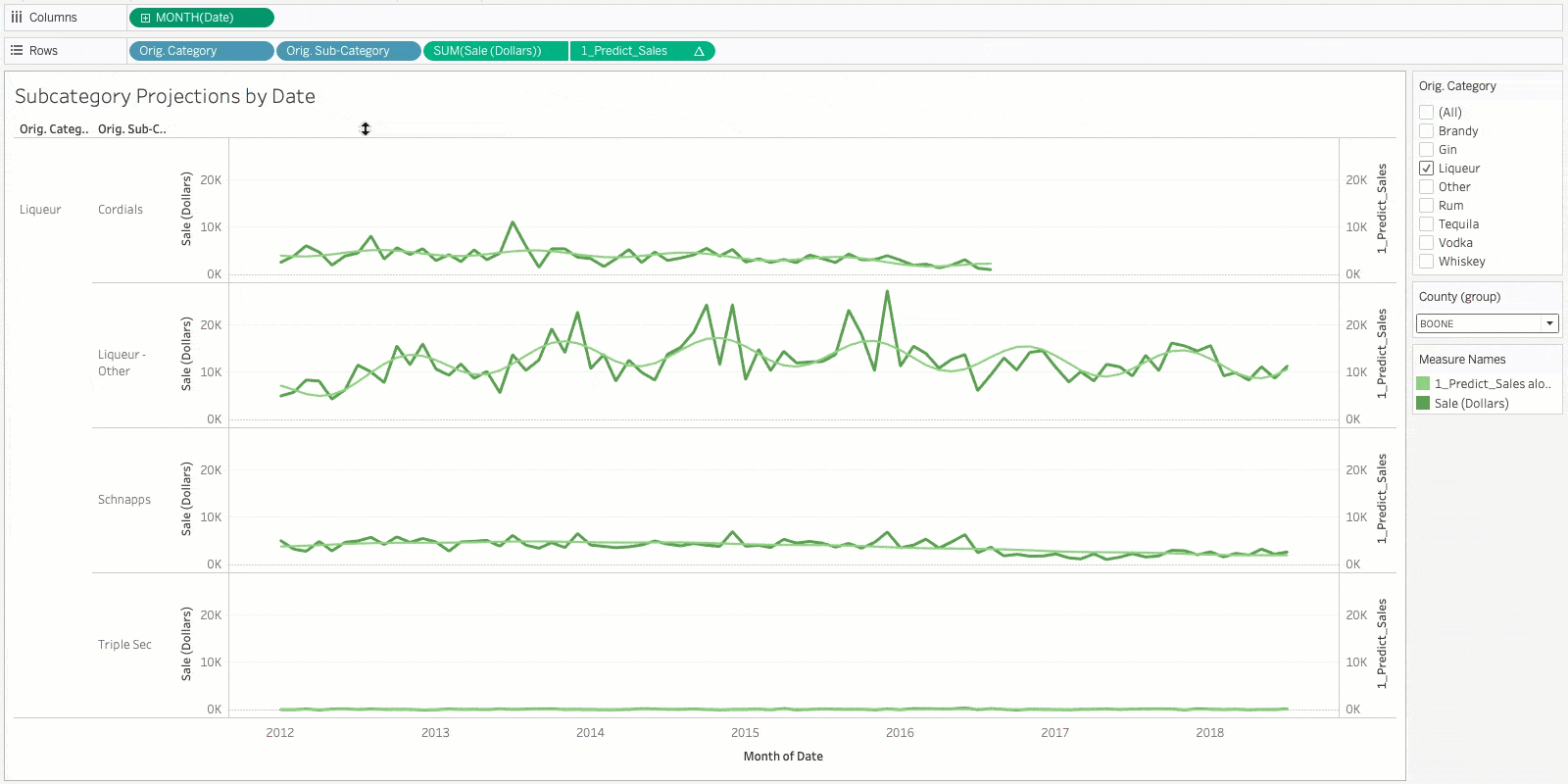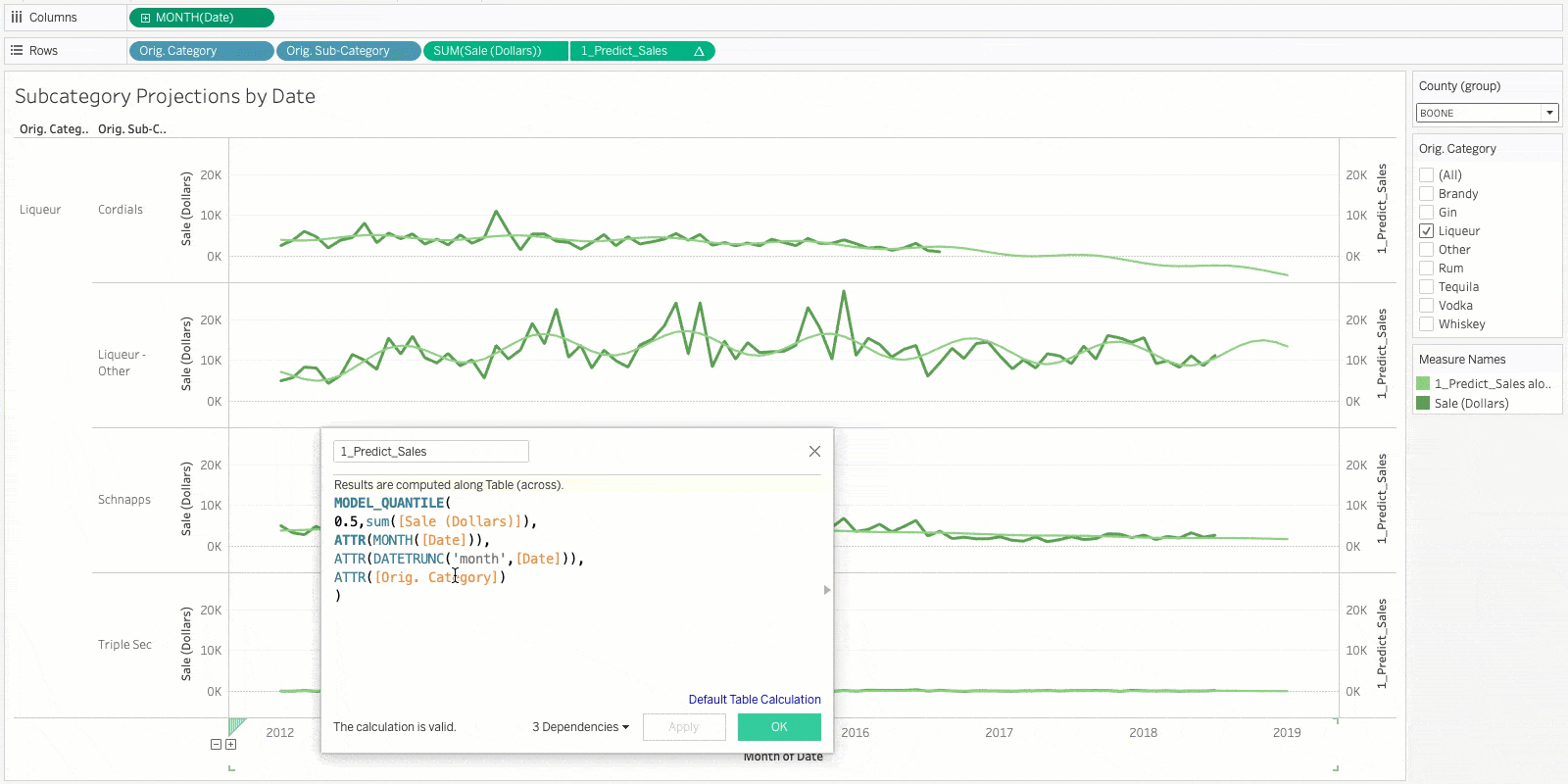# Coming soon to Tableau: More power, simplicity, and predictive flexibility

Editor's Note: Join Sarah Wachter from Tableau’s Dev team as she shares how Tableau has further improved Predictive Modeling Functions in the latest Tableau 2020.4 release. This session will introduce predictive modeling functions and demonstrate how to use them in both time-series and non-time-series applications.  Join the Dev Office Hours on Tuesday, January 26, 2021 at 12pm PT. Click here for details and registration

-

We were excited to release Predictive Modeling Functions in 2020.3, empowering Tableau users with predictive statistical functions accessible from the native Tableau table calculation interface. We put powerful predictive analytics right into the hands of business users, keeping them in the flow of working with their data. Users can quickly build statistical models and iterate based on the prediction quality, predict values for missing data, and understand relationships within their data.

However, we knew that a significant use case was still challenging. Surprising exactly no one, a key use case for predictive modeling is to generate predictions for future dates. While you can accomplish this in 2020.3 with some complicated calculations, it certainly isn’t easy.

We also knew that linear regression, specifically ordinary least squares, isn't always going to be the best predictive model for many data sets and situations. While it's very widely used and simple to understand, there are other regression models that are better suited for certain use cases or data sets, especially when you're looking at time-series data and want to make future projections.

We want to make sure that our users have the power, simplicity, and flexibility they need to apply these functions to a wide variety of use cases, and so we're delighted to announce two enhancements to predictive modeling functions. In the 2020.4 release, you'll be able to select your statistical regression model from linear regression (the default option), regularized linear regression, or Gaussian process regression. You'll also be able to extend your date range—and therefore your predictions—with just a few clicks, using a simple menu.

With these new features, Predictive Modeling Functions become even more powerful and flexible, helping you see and understand your data using best-in-class statistical techniques.

Let's take a closer look at each feature.

## Model selection

By default, predictive modeling functions use linear regression as the underlying statistical model. Linear regression is a common statistical model that is best used when there are one or more predictors that have a linear relationship with the prediction target (for example, "square footage" and "tax assessment") and those predictors don't represent two instances of the same data ("sales in GBP" and "sales in USD" represent the same data and should not both be used as predictors in a linear regression). Linear regression is suitable for a wide array of use cases, but there are some situations where a different model is better.

In 2020.4, Tableau supports linear regression, regularized linear regression, and Gaussian process regression as models. For example, regularized linear regression would be a better model in a situation where there is an approximately linear relationship between two or more predictors, such as "height" and "weight" or "age" and "salary". Gaussian process regression is best used when generating predictions across an ordered domain, such as time or space, or when there is a nonlinear relationship between the predictor and the prediction target. Models can easily be selected by including "model=linear", "model=rl", or "model=gp" as the first argument in a predictive modeling function.

## Date axis extension

Additionally, we knew that making predictions for future dates is a critical feature of predictive modeling functions. To support this, we added a new menu option to Date pills that allow you to quickly and easily extend your date axis into the future. While we built this function to support predictive modeling functions, it can also be used with RUNNING_SUM or other RUNNING_ calculations, as well as with our R & Python integrations.

Let's take a look at how these new functions can be applied!

First, let's look at how to extend your date axis and make predictions into the future. In the below example, we've already built a predictive modeling function that will predict our sales of various types of liquor. Of course, since this is a time series, we want to see what kind of sales numbers we can expect for the coming months. This is as simple as clicking the Date pill, selecting "Show Future Values", and using the menu options to set how far into the future you want to generate predictions.Next, let's look at model selection. In the below example, we've already built a predictive modeling function that uses month and category as predictors for sales of various types of liquor. We can see that the default linear regression is capturing sales seasonality and overall trends. However, we can easily switch to using regularized linear regression to see how the regularized model affects the overall amplitude of the seasonal behavior. Since we're building predictions across an ordered domain (time), Gaussian process is also a valid model to use with this data set. In either case, it's as simple as including "model=rl" or "model=gp" as the first argument of the predictive function.While we've made it very easy to switch between models, for most use cases linear regression will be an appropriate choice. Selecting an incorrect model can lead to wildly inaccurate predictions, so this functionality is best reserved for use by those with a strong statistical background and understanding of the pros and cons of different models.

## Get started with the newest version of Tableau

With these additions, we've significantly expanded the flexibility and power of our predictive modeling functions. Gaussian process regression will let you generate better predictions across a time axis, and regularized linear regression will let you account for multiple predictors being affected by the same underlying trends. Date axis extension gives you an easy, intuitive interface to generate predictions into the future, whether you're using predictive modeling functions or external services like R or Python. Look for these new features in the upcoming Tableau 2020.4 release to get started—and see what else we’re working on

As always, thank you to the countless customers and fans we've spoken with as we built these new features. We couldn't have done it without you.